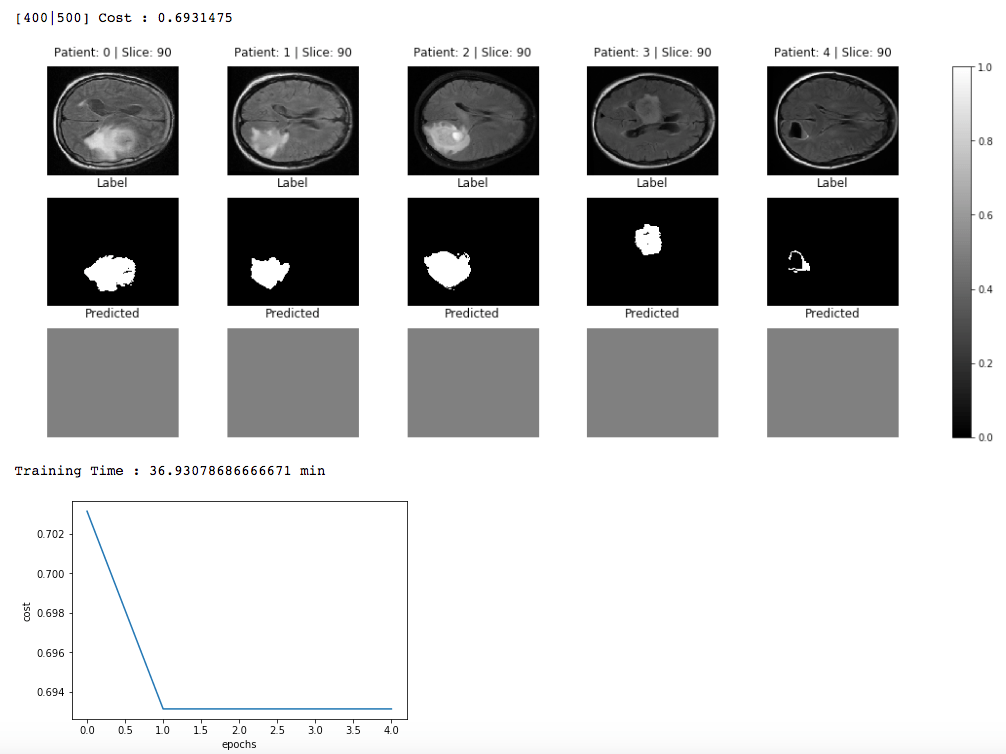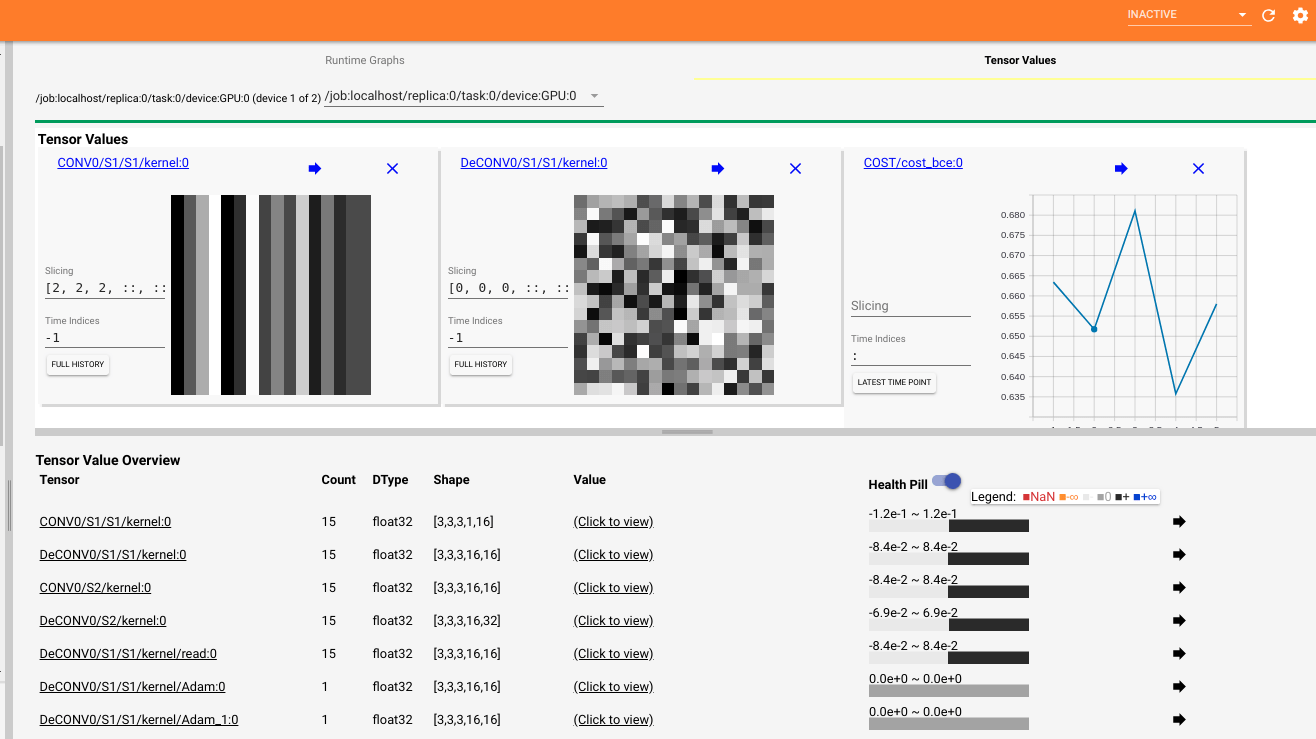# #StackBounty: #python #tensorflow #image-processing #deep-learning Tensorflow: Simple 3D Convnet not learning

### Bounty: 50

I am trying to create a simple 3D U-net for image segmentation, just to learn how to use the layers. Therefore I do a 3D convolution with stride 2 and then a transpose deconvolution to get back the same image size. I am also overfitting to a small set (test set) just to see if my network is learning but I been having trouble with it.

The cost is always 0.691475 no matter what I do (reduce learning rate, add more epochs, add more layers, change batch size…). I am sure I am doing something wrong but I can find what it is. Any help would be greatly appreciate it.

Here is my code:

``````def forward_propagation(X, patch_size, num_channels, num_labels, depth_1, mode = "train", keep_prob = 0.7):

if ( mode == 'train'): print(" --------- Net --------- ")

# Convolutional Layer 1
with tf.variable_scope('CONV1'):
Z1 = tf.layers.conv3d(X, filters = 16, kernel =[3,3,3], strides = [ 2, 2, 2], padding='SAME', name = 'S2/conv3d')
A1 = tf.nn.relu(Z1, name = 'S2/ReLU')
if ( mode == 'train'): print("Convolutional Layer 1 S2 " + str(A1.get_shape()))

# DEConvolutional Layer 1
with tf.variable_scope('DeCONV1'):
output_deconv1 = tf.stack([X.get_shape() , X.get_shape(), X.get_shape(), X.get_shape(), 1])
dZ1 = tf.nn.conv3d_transpose(A1,  filters = 1, kernel =[3,3,3], strides = [2, 2, 2], padding='SAME', name = 'S2/conv3d_transpose')
dA1 = tf.nn.relu(dZ1, name = 'S2/ReLU')

if ( mode == 'train'): print("Deconvolutional Layer 1 S1 " + str(dA1.get_shape()))

return dA1

def compute_cost(output, target, method = 'dice_hard_coe'):

with tf.variable_scope('COST'):

if (method == 'sigmoid_cross_entropy') :
# Make them vectors
output = tf.reshape( output, [-1, output.get_shape().as_list()] )
target = tf.reshape( target, [-1, target.get_shape().as_list()] )
loss = tf.nn.sigmoid_cross_entropy_with_logits(logits = output, labels = target)
cost = tf.reduce_mean(loss)

return cost
``````

and the main function for the model:

``````def model(X_h5, Y_h5, learning_rate = 0.009,
num_epochs = 100, minibatch_size = 64, print_cost = True):

ops.reset_default_graph()                         # to be able to rerun the model without overwriting tf variables
#tf.set_random_seed(1)                             # to keep results consistent (tensorflow seed)
#seed = 3                                          # to keep results consistent (numpy seed)
(m, n_D, n_H, n_W, num_channels) = X_h5["test_data"].shape   #TTT
costs = []                                        # To keep track of the cost
accuracies = []                                   # To keep track of the accuracy

# Create Placeholders of the correct shape
X, Y = create_placeholders(n_H, n_W, n_D, minibatch_size)

# Forward propagation: Build the forward propagation in the tensorflow graph
nn_output = forward_propagation(X, patch_size, num_channels, num_labels, depth_1, mode = 'train')
prediction = tf.nn.sigmoid(nn_output)

# Cost function: Add cost function to tensorflow graph
cost_method = 'sigmoid_cross_entropy'
cost = compute_cost(nn_output, Y, cost_method)

# Backpropagation: Define the tensorflow optimizer. Use an AdamOptimizer that minimizes the cost.

# Initialize all the variables globally
init = tf.global_variables_initializer()

# Start the session to compute the tensorflow graph
with tf.Session() as sess:

print('------ Training ------')

# Run the initialization
tf.local_variables_initializer().run(session=sess)
sess.run(init)

# Do the training loop
for i in range(num_epochs*m):
# ----- TRAIN -------
current_epoch = i//m

patient_start = i-(current_epoch * m)
patient_end = patient_start + minibatch_size

current_X_train = np.zeros((minibatch_size, n_D,  n_H, n_W,num_channels))
current_X_train[:,:,:,:,:] = np.array(X_h5["test_data"][patient_start:patient_end,:,:,:,:]) #TTT
current_X_train = np.nan_to_num(current_X_train) # make nan zero

current_Y_train = np.zeros((minibatch_size, n_D, n_H, n_W, num_labels))
current_Y_train = np.nan_to_num(current_Y_train) # make nan zero

feed_dict = {X: current_X_train, Y: current_Y_train}
_ , temp_cost = sess.run([optimizer, cost], feed_dict=feed_dict)

# ----- TEST -------
# Print the cost every 1/5 epoch
if ((i % (num_epochs*m/5) )== 0):

# Calculate the predictions

for j in range(0, X_h5["test_data"].shape, minibatch_size):

patient_start = j
patient_end = patient_start + minibatch_size

current_X_test = np.zeros((minibatch_size, n_D,  n_H, n_W, num_channels))
current_X_test[:,:,:,:,:] = np.array(X_h5["test_data"][patient_start:patient_end,:,:,:,:])
current_X_test = np.nan_to_num(current_X_test) # make nan zero

current_Y_test = np.zeros((minibatch_size, n_D, n_H, n_W, num_labels))
current_Y_test = np.nan_to_num(current_Y_test) # make nan zero

feed_dict = {X: current_X_test, Y: current_Y_test}
_, current_prediction = sess.run([cost, prediction], feed_dict=feed_dict)
test_predictions[j:j + minibatch_size,:,:,:,:] = current_prediction

costs.append(temp_cost)
print ("[" + str(current_epoch) + "|" + str(num_epochs) + "] " + "Cost : " + str(costs[-1]))
display_progress(X_h5["test_data"], Y_h5["test_mask"], test_predictions, 5, n_H, n_W)

# plot the cost
plt.plot(np.squeeze(costs))
plt.ylabel('cost')
plt.xlabel('epochs')
plt.show()

return
``````

I call the model with:

``````model(hdf5_data_file, hdf5_mask_file, num_epochs = 500, minibatch_size = 1, learning_rate = 1e-3)
``````

These are the results that I am currently getting:Edit:
I have tried reducing the learning rate and it doesn’t help. I also tried using tensorboard debug and the weights are not being updated:I am not sure why this is happening.
I Created the same simple model in keras and it works fine. I am not sure what I am doing wrong in tensorflow.

Get this bounty!!!

This site uses Akismet to reduce spam. Learn how your comment data is processed.